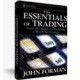Categories

# Influences on Price/Earnings Ratios

The Price/Earnings ratio (P/E) is a metric commonly used in fundamental analysis of stocks – both individually and in terms of indices. It can be a useful gauge of relative over- or under-valuation both in terms of looking at a stock or index singularly, or in comparison with others. For example, one could evaluate where the current P/E of JPM is in terms of it’s historical levels and/or in terms of how it compares to BAC, C, WFC, and others in the banking sector.

It’s not recommended that P/E be used in isolation – meaning low P/E = cheap stock, or vice versa. There are reasons why a P/E can be low or high, including changing expectations for growth rates which have not necessarily started showing up in the earning data. For that reason, you should only use the P/E in conjunction with other forms of analysis.

Looking at Stock Valuation Math
In thinking about doing so, it’s worth noting the two mathematical influences on the P/E ratio when looking at the valuation of a stock. They are the earnings growth rate and the interest rate. Stock valuations are done by determining what future earnings are expected to be, then discounting them back to the present by doing a Present Value (PV) calculation.

Earnings growth rate assumptions obviously factor into the expectations for future annual earnings per shareÂ figures. The table below shows the impact of different levels of growth rate expectations for earnings on valuation, and thus P/E.The above calculations only go out to 5 years. Valuations are often done with an additional perpetual growth rate for the years beyond #5. For the purposes here, however, five years is enough to make the point.

Notice in the yellow Value row how the valuation of the stock in question (based on adding the PVs of the earnings forecasts for Years 1 through 5) rises as the assumed annual growth rate (left column) goes from 0% up to 20%.Â Using the YearÂ 0 earningsÂ (current year achieved result) as theÂ E in the P/E, and the valuation as the P, we get the P/E listed in the right-most column. Notice how it rises in line with rising growth rates.

Now, this probably won’t come as a big surprise. It’s commonly understood that higher earningsÂ growth rates translate to higher P/Es. That’s why the P/E of a perceived growth stock will generally be higher than the P/E of a more mature stock, like a utility. It also should be noted, however, that P/Es also vary because of interest rates. The discounting of future earning’sÂ done in the valuation process employs an interest rate to calculate the PVs. Thus, interest rates impact P/Es.

The chart below provides an example.The chart above shows the P/E value of a stock with a 5% annualized earnings growth rate with valuations determined using discount rates from 1% to 10th. Notice the steady decline as interest rates rise. It’s not a big change, of course. Changes in earnings growth rates are more impactful. This may be something very important for the stock market moving forward, however. If we think interest rates are going to be rising in the years ahead, then we have to factor in slightly lower P/E ratios.## By John

Author of The Essentials of Trading